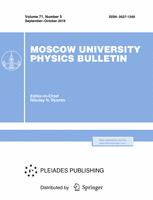Faculty of Physics
M.V.Lomonosov Moscow State University

# Investigation of magnetoresistance mechanisms of Bi$_{1.1-x}$Sn$_x$Sb$_{0.9}$Te$_2$S topological insulator

## T. N. Enderova, V. O. Sakhin, Yu. I. Talanov

### Memoirs of the Faculty of Physics 2023. N 4.

• Article
Annotation

The magnetoresistance mechanisms of Bi$_{1.1-x}$Sn$_x$Sb$_{0.9}$Te$_2$S (x = 0.02 and 0.04) topological insulators were investigated. The studied crystals have suppressed bulk conductivity, which al-lows us to study the low temperature effects that occur in the surface conducting states. In the measurements by four-probe DC resistance measurements the quantum correction improving conductivity in low fields (H > 1 kOe) was detected. This quantum correction corresponds to the case of weak antilocalization. The parameter of the phase coherence length 𝑙ϕ was extracted and its temperature dependence was determined. It was found that an external magnetic field (H > 1 kOe) activation gap appears in the energy spectrum of surface current carriers in Bi$_{1.1-x}$Sn$_x$Sb$_{0.9}$Te$_2$S (x = 0.02 and 0.04) TI. The energy of the activation gap was estimated and its dependence on the stannum concentration and the applied magnetic field was determined: 𝛥 of the order of 2 meV for Bi$_{1.06}$Sn$_{0.04}$Sb$_{0.9}$Te$_2$S and 60 μeV for Bi$_{1.08}$Sn$_{0.02}$Sb$_{0.9}$Te$_2$S at H = 5 kOe.

Approved: 2023 August 1
PACS:
72.10.Fk Scattering by point defects, dislocations, surfaces, and other imperfections
72.15.Gd Galvanomagnetic and other magnetotransport effects
72.15.Rn Localization effects
Authors
T. N. Enderova, V. O. Sakhin, Yu. I. Talanov
$^1$Zavoisky Physical-Technical Institute, FRC Kazan Scientific Center of RAS### Moscow University Physics Bulletin### Science News of the Faculty of Physics, Lomonosov Moscow State University

This new information publication, which is intended to convey to the staff, students and graduate students, faculty colleagues and partners of the main achievements of scientists and scientific information on the events in the life of university physicists.